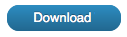# Example:- Rectangular column – ultimate strength with biaxial bending

Accompanying this is a file called pca112.xsf. It is in inch-pound units.This describes an example to illustrate the use of the application with rectangular column and biaxial bending in inch-pound units.

This example was set out in a book entitled “Notes on ACI318-77 building code requirements for reinforced concrete with design applications” published by the Portland Cement Association 1987. It was example 11.2 in that book.

That book describes the selection of a section using Design Aid SP17A and then the checking of that section for biaxial loading strength using two alternative methods. Here, that same section is checked using this application.

The cross-section input to the application followed as closely as possible the description given in the book. The concrete was 32 in. by 48 in. and the reinforcing was placed so that “gamma” was 0.9 in both directions.

The description in the book said that the reinforcing was equally distributed in all faces and that there were 30 #11 bars. This was interpreted to mean that there was one bar in each corner, five others along the shorter sides and eight other bars along each of the longer sides.

Here one parameter of strength was chosen to be the output of the computations: that one value could then be compared with a corresponding value for the same parameter computed from the requirements. The chosen parameter was the magnitude of the ultimate strength bending moment.

The requirements of the section included that:

Pn    =    2429 k

Mnx    =    4142 ‘k

Mny    =    1714 ‘k

These two bending moments are viewed as two components of one bending moment:

Magnitude    =    4483 ‘k

Orientation    =    22.48 degrees from the X axis.

During the use of the program this orientation angle, 22.48 degrees, was input as the reference angle. The axial load was set to 2429 k and the program used to compute the ultimate strength bending moment. That bending moment, from the analysis of the cross-section, could then be compared with the required bending moment.

The Curvature about the other axis restrained checkbox was not checked so that the resulting bending moment would have an orientation coinciding with the reference angle.

The example file, PCA112.XSF, includes two load cases; one called “Ultimate computed” and the other “Ultimate displayed“.

The “Ultimate computed” case gives the results as computed. It can be seen that the Moment about the other axis is negligible, and that about the reference axis is 55.765 x 103 k.in.

55.765 x 103 / 12.0    =    4647 ‘k

The 4647 ‘k is greater than the required 4483 ‘k indicating that the cross-section is adequate.

The other load case, “Ultimate displayed“, was copied from “Ultimate computed“. The computation method was changed to Utility-curvatures-and-one-strain and the orientation angle set to zero. On the Compute … dialog the Set-input-to-current-case button was pressed and then the compute button pressed.

This caused same ultimate strength situation to be described in terms of the X and Y axes. It can be seen from this that the components of the bending moment were:

Mx    =    51.561 x103 k.in     =    4297 ‘k

My    =    21.319 x103 k.in     =    1777 ‘k

As expected each of these is greater than the requirements.